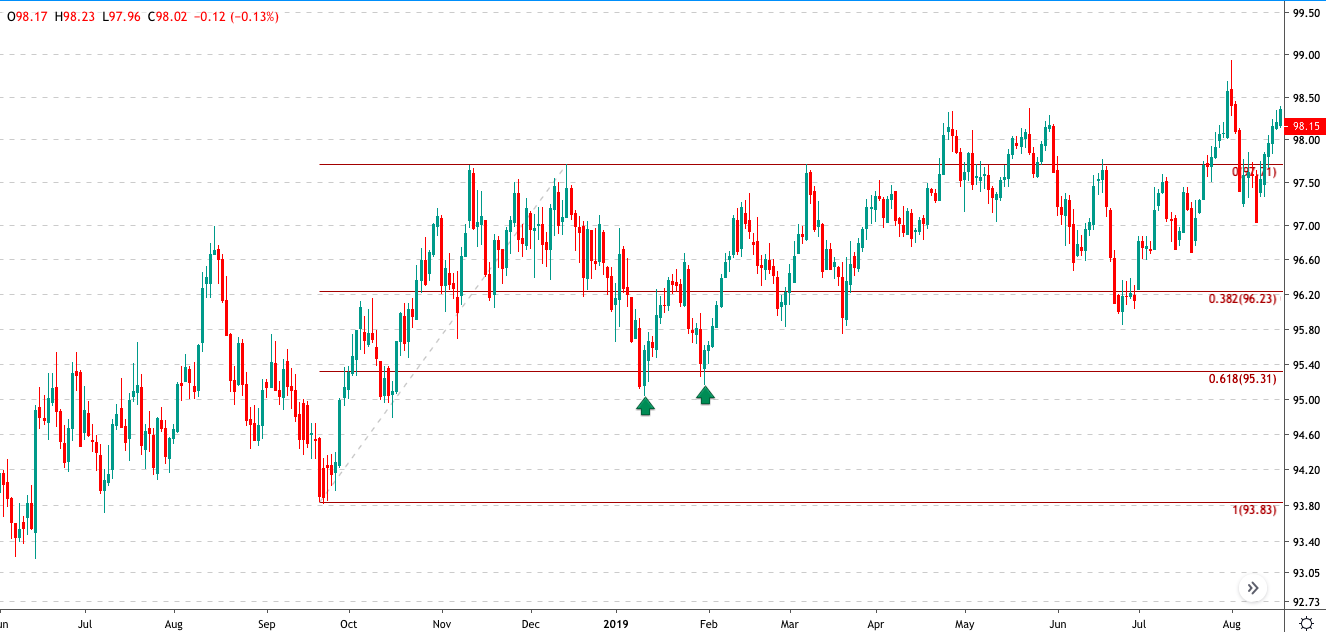# How Does Fibonacci Work In Forex ?

The term Fibonacci in forex trading terms might evoke a sense of mystery. This can be especially true if you’ve just come across it for the very first time.
But, despite the mystery, the fact is that Fibonacci-based forex trading is actually very popular.
Traders use Fibonacci methods in a number of different ways. As a result, this has given way to different technical forex trading tools with a basis in the Fibonacci numbers.
In fact, aside from using Fibonacci levels directly, there are other means of technical analysis that rely heavily on Fibonacci levels that are also quite popular. These include wave counting methods such as Elliott Waves, as well as Harmonic patterns.
So far, there aren’t any academic studies that prove that Fibonacci levels are mystical. The simplest explanation is that Fibonacci levels work as a self-fulfilling prophecy. In other words, if enough market participants start watching the same levels, there is a good chance that these levels will begin to work.
In this article, we won’t get into the details such as the origins of Fibonacci, but rather look at the different ways forex traders use Fibonacci in their approach.
Different Ways to Use Fibonacci Methods in Trading
First and foremost, using Fibonacci levels as horizontal support and resistance levels are the most widely used techniques.
In this method, FX traders measure the recent wave. By connecting the wave’s highs and lows, the Fibonacci levels are drawn automatically.
Different forex traders have their own concept of Fibonacci levels. But the most widely used are the 38.2% and 61.8% retracement levels. If you pull up any FX chart and use the Fibonacci tool, you will almost always find some market interaction taking place at these levels.
The chart below illustrates this point. We select a random small wave within the trend and then we use the Fibonacci tool. You can see how price briefly hits the 61.8% retracement level which indicates support. Following this, price then bursts higher.Fibonacci Support & Resistance
Other methods involve using Fibonacci speed resistance lines. The speed resistance lines is an old concept of technical analysis. But this is modified when you bring in the Fibonacci numbers into the picture.
The basis once again is the same. With the Fib levels, the general expectation is that price interacts at one of the Fibonacci levels either as support or resistance. The next chart below shows such an example of the Fib levels using the speed resistance lines.Fibonacci based speed resistance lines
Do Fibonacci Numbers Give You an Edge?
By now you should be wondering if using Fibonacci numbers give you any kind of edge in the forex markets. Finding an edge as an FX trader requires you to have a piece of information that your peers have yet to discover.
So, in this context, where the Fibonacci levels are so widely known, by definition, there is no proven edge in the markets.
Even still, because forex trading is generally unique, some FX traders swear by Fibonacci levels.
For example, while the 200-day or 50-day moving averages are widely used in the markets, some FX traders prefer different periods. Fibonacci numbers rounded up such as 39-period of 62-periods, can be applied to moving averages, for example.
While the numbers merely change the sensitivity and slope to the market prices, they don’t quite give you an edge that will put you in the front seat.
The topic of Fibonacci numbers is, of course, highly debatable, especially in the forex trading community. For some FX traders, Fibonacci numbers are magic. For the rest, they might not yield the same results.